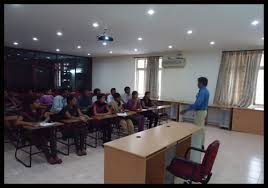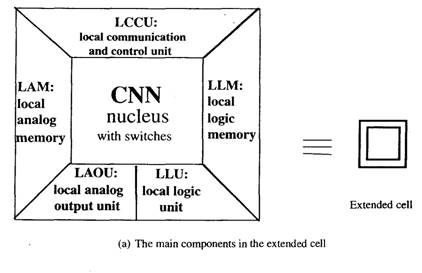## Cellular Neural Network

Published on Jan 03, 2023

### Abstract

Cellular Neural Network is a revolutionary concept and an experimentally proven new computing paradigm for analog computers. Looking at the technological advancement in the last 50 years ; we see the first revolution which led to pc industry in 1980's, second revolution led to internet industry in 1990's cheap sensors & mems arrays in desired forms of artificial eyes, nose, ears etc.

this third revolution owes due to C.N.N.This technology is implemented using CNN-UM and is also used in imageprocessing.It can also implement any Boolean functions.

### ARCHITECTURE OF CNN

A standard CNN architecture consists of an m*n rectangular array of cells c(i,j) with Cartesian coordinates (i,j) i=1,2…..M, j=12…...N.

A class -1 m*n standard CNN is defined by a m*n rectangular array of cells cij located at site (i,j) i= 1,2 …….m ,j=1,2,….n is defined mathematically by

(dXij/dt )= -Xij + A(I,j,k,l) Ykl + B(i,j,k,l) + Zij

### Simplical CNN

Recently a novel structure has been introduced to implement any Boolean / gray level function of any number of variables .The output is no longer restricted to be binary so that CNNs with gray scale outputs are obtained. Simplical CNNs are implemented using RTDs (resonant tunneling diodes)

RTDs are nano electric quantum device is featuring high speed regims and small integration sizes and they can be designed to operate in nano /femto sizes leading to extreme low power designs. In addition they exhibit an intrinsic non linear behaviour which can be exploited in many diverse applications, for instance in frequency multiplier and parity generators the threshold logic gates multi gigahertz a-d converters, and multivalued logic applications including multivalued memory design among others.

A simplical partition is used to subdivide the domain in to convex regions called simplices which are the natural extension 2-d triangle into an n-d space the corners of these simplices are called vertices & for the particular chosen domain are the points of the form ( +1,-1,+1,-1) it was proven that the set of all PWL function f is a linear vector space. Every PWL function can be expressed as a linear combination

F= € CiAi (u)

Ai(v-j)=1, if i=j

### C.N.N. Universal Machine

The first spatio temporalanalogic array computer is CNN universal machine. The two different operations are continous-time, continues valued spatio-temporal nonlinear array dynamics (2-d\$3-darrays) local & global logic hence analog &logic operations are mixed and embedded in array computer. Therefore we call this type of computing: analogic.

### The CNN-UM architecture

• It has a minimum number of component types

• Provides stored programmability spatio temporal array computing

• Is universal in two sense:

As spatial logic, it is equivalent to a Turing machine and as a local logic it may compute any Boolean function.As a nonlinear dynamic operator, it can realize any local operator of fading memory. the CNN UM is a common computational paradigm for as diverse fields of spatio-temporal computing as for example, retinal model, reaction diffusion equations, mathematical morphology, etc. The stored program ,as a sequence of templates is considered as a genetic code of CNN UM. The elementary genes are the templates.

 Are you interested in this topic.Then mail to us immediately to get the full report. email :- contactv2@gmail.com